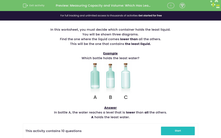# Measuring Capacity and Volume: Which Has Least?

In this worksheet, students look at containers and decide which ones hold less liquid.Key stage:  KS 1

Curriculum topic:   Measurement

Curriculum subtopic:   Solve Measurement Problems

Difficulty level:#### Worksheet Overview

In this activity are going to decide which container holds the least liquid.

Here we have three diagrams.

Let's find the one where the liquid comes lower than all the others.

This will be the one that contains the least liquid.

Example

Which bottle holds the least water?A B C